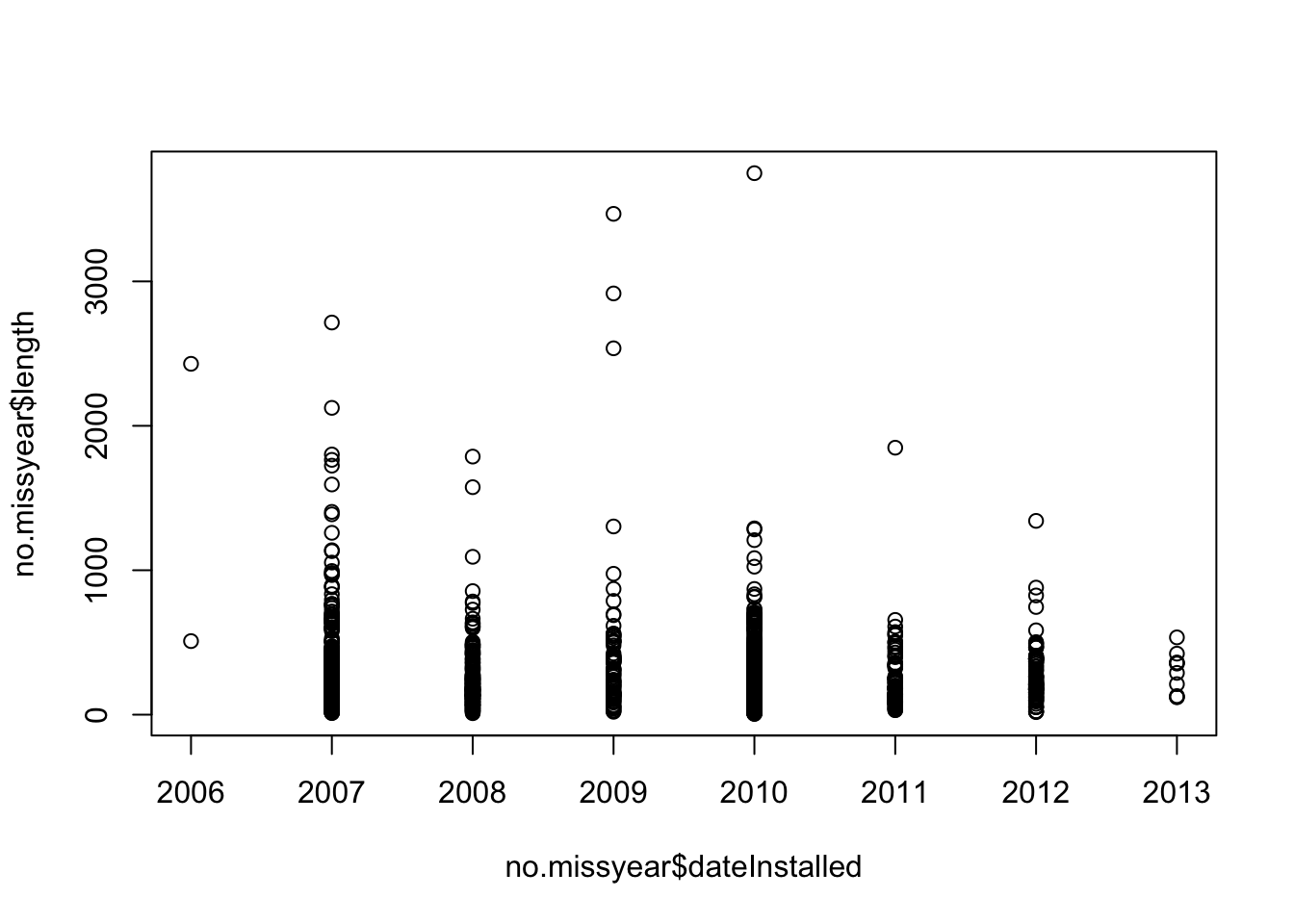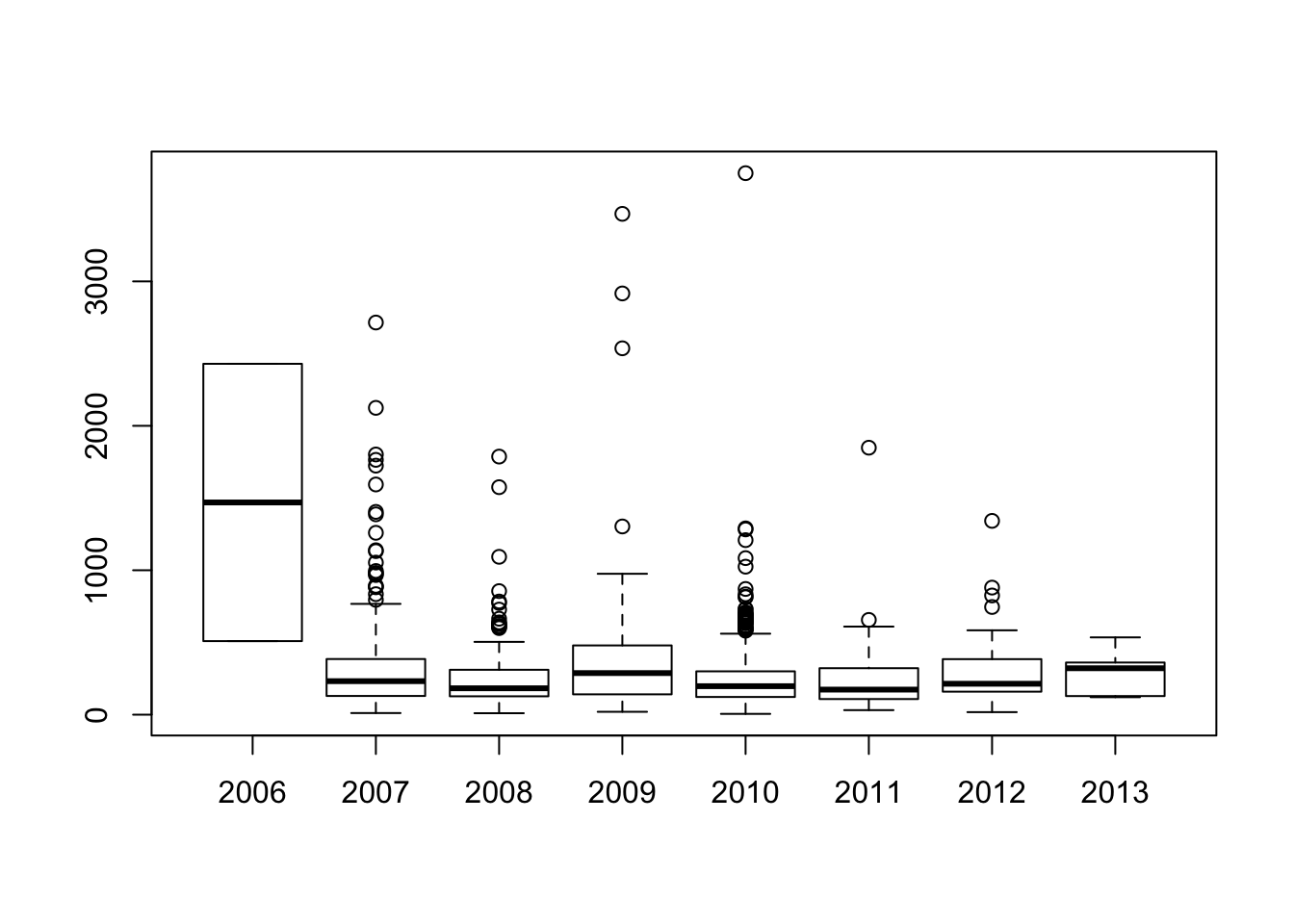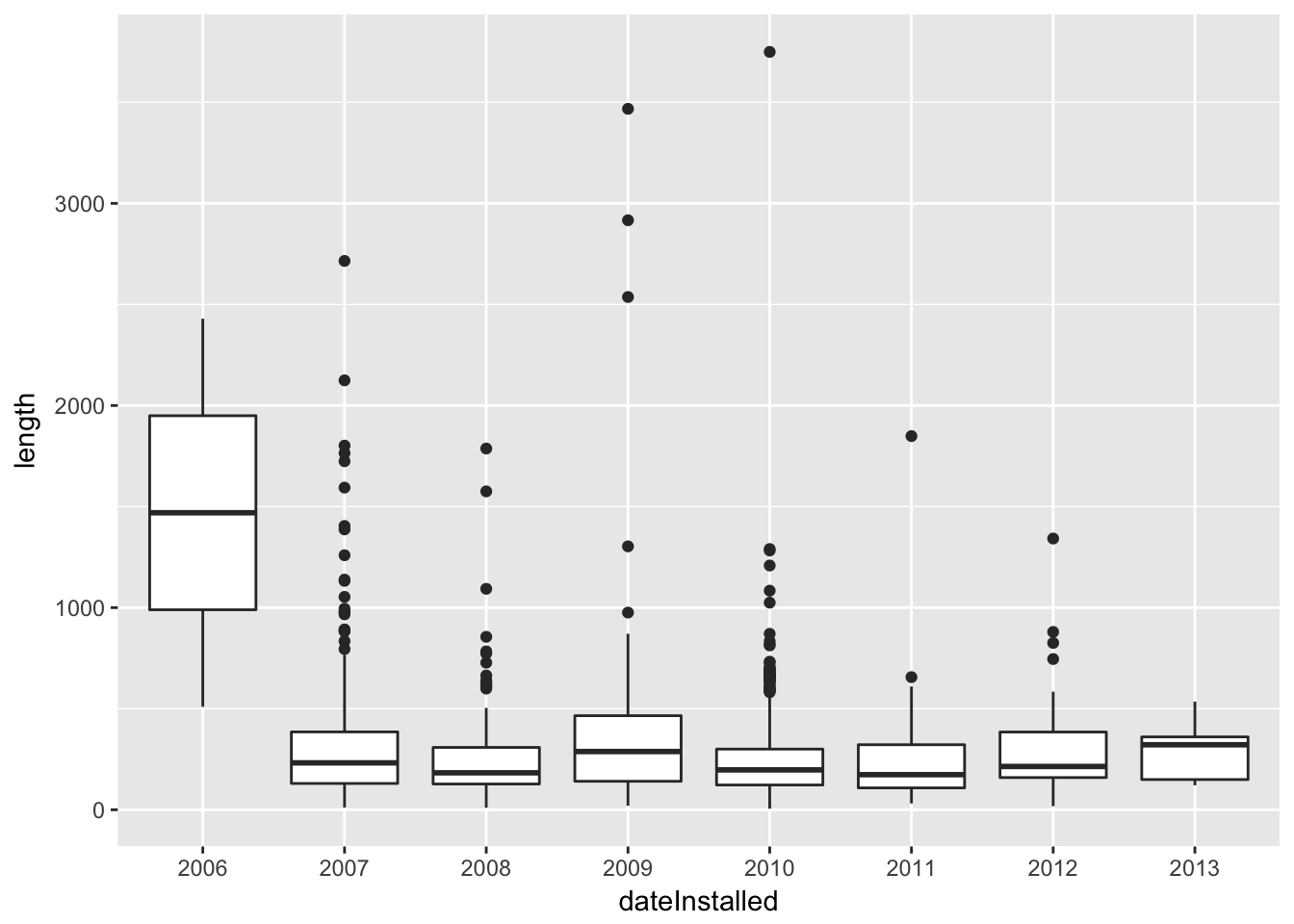The three “back ticks” (`) must be followed by curly brackets “{”, and then “r” to tell the computer that you are using R code. This line is then closed off by another curly bracket “}”.

Anything before three more back ticks “```” are then considered R code (a script).

If any code in the document has just a backtick ` then nothing, then another backtick, then that word is just printed as if it were code, such as `hey`.

I’m reading in the bike lanes here.

``````# readin is just a "label" for this code chunk
## code chunk is just a "chunk" of code, where this code usually
## does just one thing, aka a module
### comments are still # here
### let's say we loaded some packages
library(stringr)
library(dplyr)
library(tidyr)
fname <- "http://johnmuschelli.com/intro_to_r/data/Bike_Lanes.csv"

You can write your introduction here.

## Introduction

Bike lanes are in Baltimore. People like them. Why are they so long?

## Exploratory Analysis

Let’s look at some plots of bike length. Let’s say we wanted to look at what affects bike length.

### Plots of bike length

Note we made the subsection by using three “hashes” (pound signs): ###.

We can turn off R code output by using `echo = FALSE` on the knitr code chunks.``````no.missyear = no.missyear %>%  mutate(dateInstalled = factor(dateInstalled))
library(ggplot2)
gbox = no.missyear %>% ggplot(aes(x = dateInstalled, y = length)) + geom_boxplot()
print(gbox)``````We have a total of 1505 rows.

What does it look like if we took the log (base 10) of the bike length:

``````no.missyear <- no.missyear %>% mutate(log.length = log10(length))
### see here that if you specify the data argument, you don't need to do the \$
boxplot(log.length ~ dateInstalled, data = no.missyear,
main = "Boxplots of Bike Lenght by Year",
xlab="Year",
ylab="Bike Length")``````